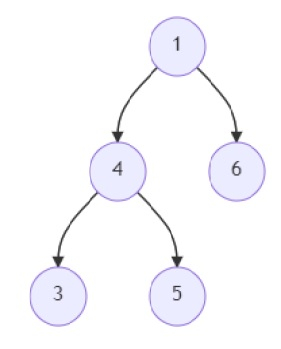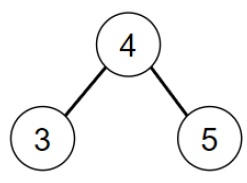# Program to find out the largest sum value of a BST in a given binary tree in Python

Suppose we are provided a binary tree. We have to find out if there exist binary search trees (BST) in the subtrees of it and find out the sum of the largest BST. To find out the sum, we add the values of each node in that BST. We return the sum value as output.

So, if the input is likethen the output will be 12.

The BST in the given binary tree is −sum of the nodes = 12.

To solve this, we will follow these steps −

• c := 0
• m := null
• value := 0
• Define a function recurse() . This will take node
• if node is not null, then
• left_val := recurse(left of node)
• right_val := recurse(right of node)
• count := negative infinity
• if (node.left is same as null or node.left.val <= node.val) and( (right of node is same as null or node.val <= node.right.val), then
• count := left_val + right_val + 1
• if count > c, then
• c := count
• m := node
• return count
• return 0
• Define a function calculate_sum() . This will take root
• if root is not same as null, then
• calculate_sum(left of root)
• value := value + value of root
• calculate_sum(right of root)
• recurse(root)
• calculate_sum(m)
• return value

## Example

Let us see the following implementation to get better understanding −

class TreeNode:
def __init__(self, val, left = None, right = None):
self.val = val
self.left = left
self.right = right

def insert(temp,data):
que = []
que.append(temp)
while (len(que)):
temp = que
que.pop(0)
if (not temp.left):
if data is not None:
temp.left = TreeNode(data)
else:
temp.left = TreeNode(0)
break
else:
que.append(temp.left)
if (not temp.right):
if data is not None:
temp.right = TreeNode(data)
else:
temp.right = TreeNode(0)
break
else:
que.append(temp.right)

def make_tree(elements):
Tree= TreeNode(elements)
for element in elements[1:]:
insert(Tree, element)
return Tree

def solve(root):
c, m, value = 0, None, 0
def recurse(node):
if node:
nonlocal c, m
left_val = recurse(node.left)
right_val = recurse(node.right)
count = -float("inf")
if (node.left == None or node.left.val <= node.val) and (node.right == None or node.val <= node.right.val):
count = left_val + right_val + 1
if count > c:
c = count
m = node
return count
return 0
def calculate_sum(root):
nonlocal value
if root is not None:
calculate_sum(root.left)
value += root.val
calculate_sum(root.right)
recurse(root)
calculate_sum(m)
return value

tree = make_tree([1, 4, 6, 3, 5])
print(solve(tree))

## Input

tree = make_tree([1, 4, 6, 3, 5])
print(solve(tree))

## Output

12